• 静态库的创建 gcc -c filen.c ar -cr libname.a file1.o file2.o ... ar：静态函数库创建的命令 -c :create的意思 -r :replace的意思，表示当前插入的模块名已经在库中存在，则替换同名的模块。如果若干模块中有一...
在Linux系统中：
静态库的创建
gcc -c filen.c ar -cr libname.a file1.o file2.o ... ar：静态函数库创建的命令 -c :create的意思 -r :replace的意思，表示当前插入的模块名已经在库中存在，则替换同名的模块。如果若干模块中有一个模块在库中不存在，ar显示一个错误信息，并不替换其他同名的模块。默认的情况下，新的成员增加在库德结尾处。
动态库的创建
gcc -shared -fpic -o libname.so test1.c test2.c .... -fpic：产生代码位置无关代码
-shared 生成一个共享库
Makefile创建库文件
比较容易懂得方式是：

LIB_NAME?= test
STATIC_NAME ?= lib$(LIB_NAME).a SHARE_NAME ?= lib$(LIB_NAME).so

all: static_library shared_library

static_library:
gcc -c *.c;
ar  -cr $(STATIC_NAME) *.o; shared_library: gcc -shared -fpic -o$(SHARE_NAME) *.c;

clean:
rm -rf *.o
rm -rf *.a *.so

上面的?= 表示如果该变量没有赋值，则进行负责操作。
下面有种比较方便管理但是比较难理解的书写方式：

LIB_NAME?= test
STATIC_NAME ?= lib$(LIB_NAME).a SHARE_NAME ?= lib$(LIB_NAME).so

all:$(STATIC_NAME)$(SHARE_NAME)

%.o:%.c
$(CC) -c$< -o $@ SOURCE :=$(wildcard *.c)
OBJS   := $(patsubst %.c,%.o,$(SOURCE))

$(STATIC_NAME):$(OBJS)
$(AR) -cr$(STATIC_NAME)

$(SHARE_NAME):$(OBJS)
gcc -shared -fpic -o $(SHARE_NAME)$(SOURCE)

clean:
rm -rf $(OBJS)$(STATIC_NAME) $(SHARE_NAME) 现在有下面的文件： . ├── include │ ├── test1.h │ ├── test2.h │ └── test3.h ├── lib ├── main.c ├── Makefile └── source ├── Makefile ├── Makefile1 ├── test1.c ├── test2.c └── test3.c └── test3.o 文件里面内容与《Makfile 应用进阶实例》中内容一致 将目录source 文件打包成一个动态库和一个静态库，随后将库文件安装到lib目录，调用的是source 目录下的Makefile Makefile: LIB_NAME?= test STATIC_NAME ?= lib$(LIB_NAME).a
SHARE_NAME  ?= lib$(LIB_NAME).so all: static_library shared_library static_library: gcc -c *.c; ar -cr$(STATIC_NAME) *.o;

shared_library:
gcc -shared -fpic -o $(SHARE_NAME) *.c; install: mv$(STATIC_NAME) ../lib
mv $(SHARE_NAME) ../lib clean: rm -rf *.o rm -rf$(STATIC_NAME) $(SHARE_NAME) 运行结果： biao@ubuntu:~/test/Makefile_test/source$
biao@ubuntu:~/test/Makefile_test/source$biao@ubuntu:~/test/Makefile_test/source$ make
gcc -c *.c;
ar  -cr libtest.a *.o;
gcc -shared -fpic -o libtest.so *.c;
biao@ubuntu:~/test/Makefile_test/source$make install mv libtest.a ../lib mv libtest.so ../lib biao@ubuntu:~/test/Makefile_test/source$ cd ../
biao@ubuntu:~/test/Makefile_test$tree . ├── include │ ├── test1.h │ ├── test2.h │ └── test3.h ├── lib │ ├── libtest.a │ └── libtest.so ├── main.c ├── Makefile └── source ├── Makefile ├── Makefile1 ├── test1.c ├── test1.o ├── test2.c ├── test2.o ├── test3.c └── test3.o 3 directories, 15 files biao@ubuntu:~/test/Makefile_test$

库文件应用：
如果直接在shell中使用命令：
动态调用库文件：
gcc  main.c -I./include/ -L./lib/ -ltest -o app在运行可执行文件APP之前，需要指定动态库的位置，否则会出现找不到库文件的错误： export LD_LIBRARY_PATH='/home/biao/test/Makefile_test/lib/' 如果不指定库的位置，也可以直接将库放到/lib 或者/usr/lib下
静态库文件调用：
gcc -static  main.c -I./include/ -L./lib/ -ltest -o app_static   另一种方法：直接添加静态库 gcc  main.c -I./include/ ./lib/libtest.a -o app_static 如果同时有静态库和动态库，默认使用的是动态库。
在Makefile中链接库文件：

EXE?= test
SHARE_EXE  ?= share_$(EXE) STATIC_EXE ?= static_$(EXE)

INCL := -I./include/
LIB  := -L./lib/
LIB_NAME := test
FLAG := -static

all: static_app shared_app

static_app:
$(CC)$(FLAG)  main.c $(INCL)$(LIB) -l$(LIB_NAME) -o$(STATIC_EXE)

shared_app:
$(CC) main.c$(INCL) $(LIB) -l$(LIB_NAME) -o $(SHARE_EXE) clean: rm -rf$(STATIC_EXE) $(SHARE_EXE) 这里同时生产两个可执行文件：share_test 和 static_test 执行结果如下： biao@ubuntu:~/test/Makefile_test$
biao@ubuntu:~/test/Makefile_test$ls include lib main.c Makefile source biao@ubuntu:~/test/Makefile_test$ make
cc -static  main.c -I./include/ -L./lib/ -ltest -o static_test
cc  main.c -I./include/ -L./lib/ -ltest -o share_test
biao@ubuntu:~/test/Makefile_test$ls include lib main.c Makefile share_test source static_test biao@ubuntu:~/test/Makefile_test$ ./share_test
I am test1 !
I am test2 !
I am test3 !
biao@ubuntu:~/test/Makefile_test$./static_test I am test1 ! I am test2 ! I am test3 ! biao@ubuntu:~/test/Makefile_test$
biao@ubuntu:~/test/Makefile_test$ 测试代码可以到这里下载： Linux Makefile 静态库动态库应用实例 展开全文动态库 • # 使用ar链接生成静态库$(LIB_DIR)/libmylib.a:$(LIB_OBJ) ar -crv$@ $^$(OBJ_DIR)/%.o:src/%.c $(LIB_CC) -c$$@ # 使用静态库 test:a.out a.out:test.c$(LIB_CC) test.c -L$(LIB_DIR) -lmylib clean: ... mkdir testlib,组织文件目录如下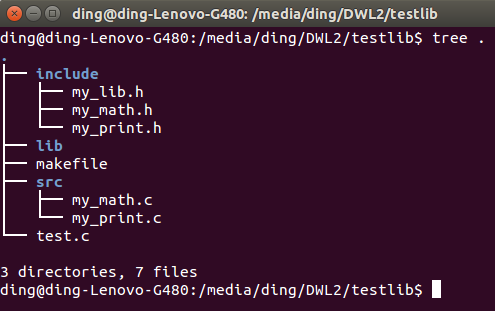my_math.h int add(int a, int b); int subtract(int a, int b); my_math.c #include "../include/my_math.h" int add(int a, int b) { return a + b; } int subtract(int a, int b) { return a - b; } my_print.h #include <stdio.h> void cout(const char * message);my_print.c #include "../include/my_print.h" void cout(const char * message) { fprintf(stdout, "%s\n", message); } mylib.h #ifndef __MY_LIB_H__ #define __MY_LIB_H__ int add(int a, int b); int subtract(int a, int b); void cout(const char *); #endif 有以上文件 生成 libmylib.a 编写测试文件test.c，引用libmylib.a test.c #include <stdio.h> #include "my_lib.h" int main(void) { int c = add(15, -21); cout("I am a func from mylib ..."); printf("%d\n",c); return 0; } makefile # 静态库的使用 # # make build 生成 libmylib.a # make test 生成a.out可执行文件 # make clean 清除相关文件 # .PHONY: build test clean CC=gcc OBJ_DIR=./obj LIB_DIR=./lib HEADERS=-I./include DEBUG=-g -ggdb WALL=-Wall -W CFLAGS=$(WALL) $(DEBUG) LIB_CC=$(CC) $(CFLAGS)$(HEADERS)

LIB_OBJ = $(addprefix$(OBJ_DIR)/, my_math.o my_print.o)

# 目录的判断与创建
prepare:
@-if [ ! -d $(OBJ_DIR) ];then mkdir$(OBJ_DIR); fi
@-if [ ! -d $(LIB_DIR) ];then mkdir$(LIB_DIR); fi

build:prepare $(LIB_DIR)/libmylib.a # 使用ar链接生成静态库$(LIB_DIR)/libmylib.a:$(LIB_OBJ) ar -crv$@ $^$(OBJ_DIR)/%.o:src/%.c
$(LIB_CC) -c$< -o $@ # 使用静态库 test:a.out a.out:test.c$(LIB_CC) test.c -L$(LIB_DIR) -lmylib clean: rm -rf$(OBJ_DIR)
rm $(LIB_DIR)/libmylib.a rm a.out makefile相关命令： makefile中的.PHONY: http://blog.163.com/yangfan876@126/blog/static/80612456201371255834418/ shell的addprefix： http://blog.csdn.net/cjsycyl/article/details/8064958 makefile中的$^ , $@,$<作用：http://blog.csdn.net/laogaoav/article/details/8520258
linux ar命令 静态库：http://blog.csdn.net/xuhongning/article/details/6365200

执行相应的make命令后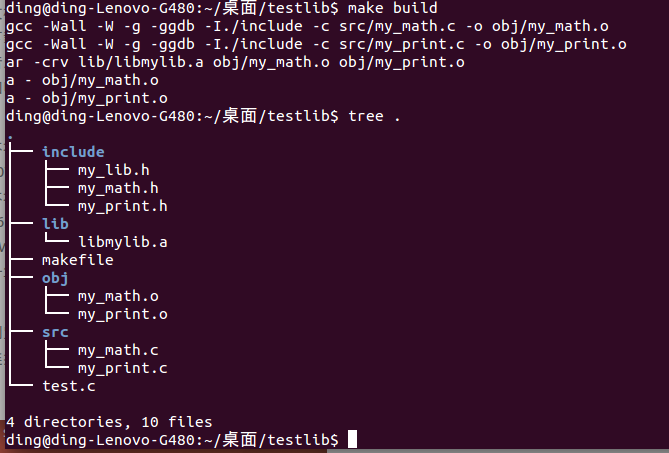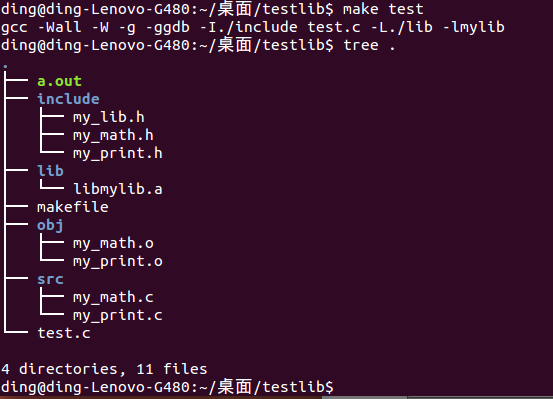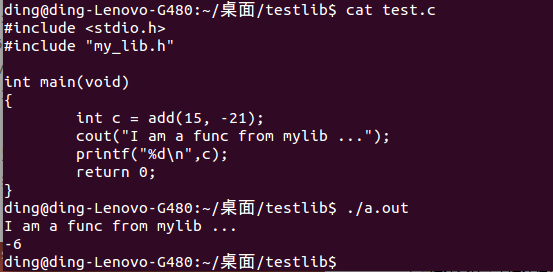参考
linux 静态库生成 http://www.cnblogs.com/jiqingwu/p/4325382.html

Linux 动态库与静态库制作及使用详解:http://blog.csdn.net/mr_chenping/article/details/23823825


展开全文• 生成Linux静态库的工程模板，只要在Makefile 文件中添加工程中源文件头文件的路径，就可以生成Linux静态库。这里是demo工程。
• 1. 静态库生成makefile命令的简介可参考：跟我一起写 Makefile。使用ar命令生成.a文件，可参考：Linux下动态库(.so)和静态库(.a)# 1、准备工作，编译方式、目标文件名、依赖库路径的定义。 CC = g++ CFLAGS := -...
根据GenDll.cpp文件，分别生成动态库.so和静态库.a文件，需要依赖的外部库为opencv。
1. 静态库的生成
makefile命令的简介可参考：跟我一起写 Makefile。使用ar命令生成.a文件，可参考：Linux下动态库(.so)和静态库(.a)
# 1、准备工作，编译方式、目标文件名、依赖库路径的定义。
CC = g++
CFLAGS  := -Wall -O3 -std=c++0x

# opencv 头文件和lib路径
OPENCV_INC_ROOT = /usr/local/include/opencv
OPENCV_LIB_ROOT = /usr/local/lib

OBJS = GenDll.o #.o文件与.cpp文件同名
LIB = libgendll.a # 目标文件名

OPENCV_INC= -I $(OPENCV_INC_ROOT) INCLUDE_PATH =$(OPENCV_INC)

LIB_PATH = -L $(OPENCV_LIB_ROOT) # 依赖的lib名称 OPENCV_LIB = -lopencv_objdetect -lopencv_core -lopencv_highgui -lopencv_imgproc all :$(LIB)

# 2. 生成.o文件
%.o : %.cpp
$(CC)$(CFLAGS) -c $< -o$@ $(INCLUDE_PATH)$(LIB_PATH) $(OPENCV_LIB) # 3. 生成静态库文件$(LIB) : $(OBJS) rm -f$@
ar cr $@$(OBJS)
rm -f $(OBJS) tags : ctags -R * # 4. 删除中间过程生成的文件 clean: rm -f$(OBJS) $(TARGET)$(LIB)
2. 动态库的生成
第1、4步准备和收尾工作与静态库的保持一致，第2步和第3步所使用的命令稍有不同。
# 1、准备工作，编译方式、目标文件名、依赖库路径的定义。
CC = g++
CFLAGS  := -Wall -O3 -std=c++0x

# opencv 头文件和lib路径
OPENCV_INC_ROOT = /usr/local/include/opencv
OPENCV_LIB_ROOT = /usr/local/lib

OBJS = GenDll.o #.o文件与.cpp文件同名
LIB = libgendll.so # 目标文件名

OPENCV_INC= -I $(OPENCV_INC_ROOT) INCLUDE_PATH =$(OPENCV_INC)

LIB_PATH = -L $(OPENCV_LIB_ROOT) # 依赖的lib名称 OPENCV_LIB = -lopencv_objdetect -lopencv_core -lopencv_highgui -lopencv_imgproc all :$(LIB)

# 2. 生成.o文件
%.o : %.cpp
$(CC)$(CFLAGS) -fpic -c $< -o$@ $(INCLUDE_PATH)$(LIB_PATH) $(OPENCV_LIB) # 3. 生成动态库文件$(LIB) : $(OBJS) rm -f$@
g++ -shared -o $@$(OBJS)
rm -f $(OBJS) tags : ctags -R * # 4. 删除中间过程生成的文件 clean: rm -f$(OBJS) $(TARGET)$(LIB)
-fpic 和 -shared 命令可参考：Linux下动态库(.so)和静态库(.a)【注】这篇文章说可以使用ld命令生成.so文件，但我在测试时发会报错。
3. 动态库和静态库的调用
， 这两个的使用方法几乎没有区别。动态库的引用有显式和隐式两种，这里只说隐式调用。我使用main.cpp来测试生成的库文件， makefile如下：
CC = g++
CFLAGS  := -Wall -O3 -std=c++0x

OPENCV_INC_ROOT = /usr/local/include/opencv
OPENCV_LIB_ROOT = /usr/local/lib
MY_ROOT = ../

OPENCV_INC= -I $(OPENCV_INC_ROOT) MY_INC = -I$(MY_ROOT)

EXT_INC = $(OPENCV_INC)$(MY_INC)

OPENCV_LIB_PATH = -L $(OPENCV_LIB_ROOT) MY_LIB_PATH = -L$(MY_ROOT)

EXT_LIB = $(OPENCV_LIB_PATH)$(MY_LIB_PATH)

OPENCV_LIB_NAME = -lopencv_objdetect -lopencv_highgui -lopencv_imgproc -lopencv_core
MY_LIB_NAME = -lgendll

all:test

test:main.cpp
$(CC)$(CFLAGS) main.cpp $(EXT_INC)$(EXT_LIB) $(MY_LIB_NAME)$(OPENCV_LIB_NAME) -o test

4. 注意事项：
1、在测试过程中，经常会报错：找不到.so文件。一种简单的解决方法如下：  在linux终端输入如下命令：
export LD_LIBRARY_PATH=/home/shaoxiaohu/lib:LD_LIBRARY_PATH:
更多解决方法可参考：Linux下gcc编译生成动态链接库*.so文件并调用它的第4部分。
—–
makefile还需要学习，有很多细节还没有很明白。现在仍没有做到活学活用，应用仅限于套模板。
展开全文so a
• 根据GenDll.cpp文件，分别生成动态库.so和静态库.a文件，需要依赖的外部库为opencv。 1. 静态库生成 makefile命令的简介可参考：跟我一起写 ...使用ar命令生成.a文件，可参考：Linux下动态库(.so)和静态库(....
（转自：https://blog.csdn.net/shaoxiaohu1/article/details/46943417）

根据GenDll.cpp文件，分别生成动态库.so和静态库.a文件，需要依赖的外部库为opencv。
1. 静态库的生成
makefile命令的简介可参考：跟我一起写 Makefile。使用ar命令生成.a文件，可参考：Linux下动态库(.so)和静态库(.a)
# 1、准备工作，编译方式、目标文件名、依赖库路径的定义。
CC = g++
CFLAGS  := -Wall -O3 -std=c++0x

# opencv 头文件和lib路径
OPENCV_INC_ROOT = /usr/local/include/opencv
OPENCV_LIB_ROOT = /usr/local/lib

OBJS = GenDll.o #.o文件与.cpp文件同名
LIB = libgendll.a # 目标文件名

OPENCV_INC= -I $(OPENCV_INC_ROOT) INCLUDE_PATH =$(OPENCV_INC)

LIB_PATH = -L $(OPENCV_LIB_ROOT) # 依赖的lib名称 OPENCV_LIB = -lopencv_objdetect -lopencv_core -lopencv_highgui -lopencv_imgproc all :$(LIB)

# 2. 生成.o文件
%.o : %.cpp
$(CC)$(CFLAGS) -c $< -o$@ $(INCLUDE_PATH)$(LIB_PATH) $(OPENCV_LIB) # 3. 生成静态库文件$(LIB) : $(OBJS) rm -f$@
ar cr $@$(OBJS)
rm -f $(OBJS) tags : ctags -R * # 4. 删除中间过程生成的文件 clean: rm -f$(OBJS) $(TARGET)$(LIB)
1234567891011121314151617181920212223242526272829303132333435363738
2. 动态库的生成
第1、4步准备和收尾工作与静态库的保持一致，第2步和第3步所使用的命令稍有不同。
# 1、准备工作，编译方式、目标文件名、依赖库路径的定义。
CC = g++
CFLAGS  := -Wall -O3 -std=c++0x

# opencv 头文件和lib路径
OPENCV_INC_ROOT = /usr/local/include/opencv
OPENCV_LIB_ROOT = /usr/local/lib

OBJS = GenDll.o #.o文件与.cpp文件同名
LIB = libgendll.so # 目标文件名

OPENCV_INC= -I $(OPENCV_INC_ROOT) INCLUDE_PATH =$(OPENCV_INC)

LIB_PATH = -L $(OPENCV_LIB_ROOT) # 依赖的lib名称 OPENCV_LIB = -lopencv_objdetect -lopencv_core -lopencv_highgui -lopencv_imgproc all :$(LIB)

# 2. 生成.o文件
%.o : %.cpp
$(CC)$(CFLAGS) -fpic -c $< -o$@ $(INCLUDE_PATH)$(LIB_PATH) $(OPENCV_LIB) # 3. 生成动态库文件$(LIB) : $(OBJS) rm -f$@
g++ -shared -o $@$(OBJS)
rm -f $(OBJS) tags : ctags -R * # 4. 删除中间过程生成的文件 clean: rm -f$(OBJS) $(TARGET)$(LIB)
1234567891011121314151617181920212223242526272829303132333435363738
-fpic 和 -shared 命令可参考：Linux下动态库(.so)和静态库(.a)【注】这篇文章说可以使用ld命令生成.so文件，但我在测试时发会报错。
3. 动态库和静态库的调用
， 这两个的使用方法几乎没有区别。动态库的引用有显式和隐式两种，这里只说隐式调用。我使用main.cpp来测试生成的库文件， makefile如下：
CC = g++
CFLAGS  := -Wall -O3 -std=c++0x

OPENCV_INC_ROOT = /usr/local/include/opencv
OPENCV_LIB_ROOT = /usr/local/lib
MY_ROOT = ../

OPENCV_INC= -I $(OPENCV_INC_ROOT) MY_INC = -I$(MY_ROOT)

EXT_INC = $(OPENCV_INC)$(MY_INC)

OPENCV_LIB_PATH = -L $(OPENCV_LIB_ROOT) MY_LIB_PATH = -L$(MY_ROOT)

EXT_LIB = $(OPENCV_LIB_PATH)$(MY_LIB_PATH)

OPENCV_LIB_NAME = -lopencv_objdetect -lopencv_highgui -lopencv_imgproc -lopencv_core
MY_LIB_NAME = -lgendll

all:test

test:main.cpp
$(CC)$(CFLAGS) main.cpp $(EXT_INC)$(EXT_LIB) $(MY_LIB_NAME)$(OPENCV_LIB_NAME) -o test

12345678910111213141516171819202122232425
4. 注意事项：
1、在测试过程中，经常会报错：找不到.so文件。一种简单的解决方法如下：  在linux终端输入如下命令：
export LD_LIBRARY_PATH=/home/shaoxiaohu/lib:LD_LIBRARY_PATH:
1
更多解决方法可参考：Linux下gcc编译生成动态链接库*.so文件并调用它的第4部分。
—–
makefile还需要学习，有很多细节还没有很明白。现在仍没有做到活学活用，应用仅限于套模板。

展开全文编译 动态库
• 引用Jesse Rei 的 linux 生成和使用动态链接静态链接Makefile编写生成和使用动态链接静态链接Makefile编写1. 概述介绍linux生成和使用动态链接静态链接Makefile的编写方法。 2. 生成动态...redhat gcc c
• Linux的Terminal中如何生成静态库以及如何使用静态库生成静态库文件分为两个步骤 hello.c#include void hello() { printf("hello\n"); }main.c#include #include"hello.h" int main() { printf("Hello main\n"); ...Terminal
• 项目引入了多个三方静态库，其中包含的.o文件重名，但是幸运的是函数不重名，类不重名，因此只需要在ar时重命名.o文件即可。 makefile中： tmp赋值为取出文件名（第一步去除目录和第二步去除后缀） makefile中for...
• 库文件源码为：app.c使用库文件源码为：main.c静态链接库生成：gcc -c app.car rcs libtest.a app.o静态库文件名的命名规范是以lib为前缀，紧接着跟静态库名，扩展名为.a。例如：我们要生成名为test的静态库，则静态...c c
• Linux下，工程管理器make是可用于自动编译、链接程序的实用工具。我们要做的是写一个Makefile... GNU autotools作用：收集系统配置信息并自动生成Makefile文件。  GNU autotools主要包括三个工具：autoconf、automaautotools
• #=============================================# Makefile (linux) for S21SDK Sample : testnc#=============================================SHELL = /bin/shCC = mipsel-linux-gccAR = mipsel-linux-arCshell
• #=============================================# Makefile (linux) for S21SDK Sample : testnc#=============================================SHELL = /bin/shCC = mipsel-linux-gccAR = mipsel-linux-arCshell
• Linux中用Makefile管理静态库与动态库 本人是程序员小白一枚，还望大家多多批评指点 先介绍一下静态库 程序在编译链接时将哭的代码链接到可行文件中。 程序运行不再需要静态库。 前情提要：静态库的缺点就是占...c语言
• 动态库、静态库LinuxC/C++软件项目常用的调用方法，在程序运行目录放入过多的静态库显得混乱，也难免由于共同依赖造成冗余。若项目中依赖关系如下图所示，则可以将静态库一层层的打包在一起。本文以简单的hello...
• 测试环境是ubuntu首先从http://www.jenkinssoftware.com/index.html 下载RakNet-3.7....tar.gz解压压缩文件并进入.../Source目录编译成静态库和动态库有两种方式1，传统的linux生成静态库和动态库方式 静态库 如果...ubuntu 测试
• 一.库 什么是库，简单的可以说是可执行代码的二进制...linux静态库（.a）文件，动态库（.so）文件。主要存放函数库的路径有：/lib , /usr/lib. 二.静态库与动态库 1.静态库 这类库的名字一般是libname.a.利用静态库
• Lunix下编译静态库文件： .o后缀文件：编译生成的中间代码文件； .a后缀文件：静态库文件，编译的时候...用一个简单的例子阐述下在lunix环境下生成静态库文件的过程： 一. 源码文件： so_test.h： voi
• 用ar归档目标文件，生成静态库。 配合静态库，写一个使用静态库中函数的头文件。 使用静态库时，在源码中包含对应的头文件，链接时记得链接自己的库。 下面通过实例具体讲解。 写源文件，生成目标文件。 第一个...
• linux下的静态库与动态库的区别 1.什么是库 在windows平台和linux平台下都大量存在着库。 本质上来说库是一种可执行代码的二进制形式，可以被操作系统载入内存执行。 由于windows和linux的本质不同，因此二者库...header output unix
• 三、使用Makefile制作动态库、静态库 3.1 Linux下的库（动态库、静态库）简介 3.1.1 什么是库 3.1.2库的种类 3.2.3 库存在的意义 3.2.4在linux下如何制作库文件 3.2.5 查看可执行程序依赖的库（ldd命令） ...
• 环境：Ubuntu-13.10 32位(虚拟机)、gcc4.8.1 首先创建一个test_makefile_gcc文件夹，此test_makefile_gcc文件夹下包括：src文件夹...lib文件夹用于存放生成静态库.a文件；project_makefile文件夹存放此工程的ma...gcc
• Makefile 一个工程中的源文件不计其数，其按类型、功能、模块分别放在若干个目录中，makefile定义了一系列的规则来指定，哪些文件需要先编译，...在 Linux（unix ）环境下使用GNU 的make工具能够比较容易的构建一个属...

# linux生成静态库makefile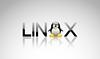linux 订阅